## Air at 20ºC with a convection heat transfer coefficient of 20 W/m2·K blows over a pond. The surface temperature of the pond is 23ºC. Determi

Question

Air at 20ºC with a convection heat transfer coefficient of 20 W/m2·K blows over a pond. The surface temperature of the pond is 23ºC. Determine the heat flux between the surface of the pond and the air. The heat flux between the surface of the pond and the air is

in progress 0
5 months 2021-08-24T12:48:21+00:00 1 Answers 1 views 0

## Answers ( )

The heat flux between the surface of the pond and the surrounding air  is 60 W/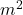Explanation:

Heat flux is the rate at which heat energy moves across a surface, it is the heat transferred per unit area of the surface. This can be calculated using the expression in equation 1;

q = Q/A ………………………….1

since we are working with the convectional heat transfer coefficient equation 1 become;

q = h (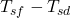) ……………………2

where q is the  heat flux;

Q is the heat energy that will be transferred;

h is the convectional heat coefficient = 20 W/.K;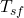is the surface temperature =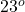C 23°C + 273.15  = 296.15 K;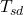is the surrounding temperature =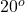C = 20°C + 273.15  = 293.15 K;

The values are substituted into equation 2;

q = 20 W/.K (  296.15 K – 293.15 K)

q = 20 W/.K ( 3 K)

q =  60 W/Therefore the heat flux between the surface of the pond and the surrounding air  is 60 W/